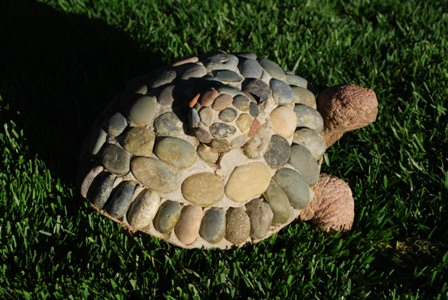## Wizard Recommends

In 2009, the total amount wagered on blackjack tables in Nevada was \$8.917 billion. The casinos won \$1.008 billion. How much of that is attributable to player mistakes?

reno

From the Nevada Gaming Control Board 2009 revenue report, we see the win for "21" was indeed \$1,008,525,000. That probably includes blackjack variants. According to my February 20, 2010 Ask the Wizard column, the cost of mistakes in blackjack is about 0.83%, according to gaming consultant Bill Zender.

The missing piece is what would be the house edge without the errors? I admit this is kind of crude, but the average of the house edge column in the April 2010 Current Blackjack Newsletter is 0.78%. So, the total house edge in blackjack, including errors is 0.78% + 0.83% = 1.61%. The portion of that due to errors is 0.83%/1.61% = 51.55%. So the 2009 profit from blackjack errors in Nevada could be roughly estimated as 1,008,525,000 × 0.5155 = \$519 million.

This question was raised and discussed in the forum of my companion site Wizard of Vegas.

What are the odds of getting a royal flush in 9-6 Jacks or Better holding one card only?

James from Spencer, MA

The following table shows the probability of each kind of royal, according to the number of cards held, given that there was a royal. It shows that 3.4% of royals are from holding one card. The probability of a royal to begin with is 1 in 40,391, so the unconditional probability of a royal holding one card is 1 in 1,186,106.

### 9/6 Jacks Royal Combinations

Cards Held Combinations Probability
0 1,426,800 0.002891
1 16,805,604 0.034053
2 96,804,180 0.196154
3 195,055,740 0.395240
4 152,741,160 0.309498
5 30,678,780 0.062164
Total 493,512,264 1.000000

In your page on Megabucks, you said the value of 25 equal annual payments at the beginning of each year, with an interest rate of 4.66%, is worth 61.07% of face value. I was wondering what formula you used for the annuity?

AZDuffman

The formula is V = P × [(1-(1+i)-n)]/(i/(1+i)), where:

V = value of annuity
P = individual payment amount
i = interest rate
n = number of payments

Let’s say the jackpot was \$15M. Using i = 4.66%, and n=25, the fair payment to keep up with inflation would be \$982,525. You would actually get 15M/25 = \$600,000. Actual payment/fair payment = 61.07%.

Not that you asked, but the formula if the payments are made at the end of each year is V = P × [(1-(1+i)-n)]/i.

This question was raised and discussed in the forum of my companion site Wizard of Vegas.

I sell sculptures. On average, out of every seven sculpture sales, one will be a turtle, and the rest will be other types of sculptures. How many turtles do I need to have in stock if I want a 90% chance of not running out in the next 100 sculpture sales?

RbStimersThis is a good confidence interval kind of problem. In 100 sales the expected turtles sold will be 14.29. The standard deviation is sqrt(100×(1/7)×(6/7)) = 3.50.

Let t be the number of turtles made, and x the number sold.

pr(x<=t)=0.9
pr(x-14.29<=t-14.29)=0.9
pr((x-14.29)/3.5)<=(t-14.29)/3.5))=0.9

The left side of the inequality follows a standard normal distribution (mean of 0, standard deviation of 1). This next step takes an introductory statistics course, or some faith, to accept.

(t-14.29)/3.5 = normsinv(0.9) This is the Excel function.
(t-14.29)/3.5 = 1.282
t-14.29 = 4.4870
t = 18.77

Nobody is likely to buy 0.77 of a turtle statue, so I would round up to 19. According to the binomial distribution, the probability of selling 18 or less is 88.35%, and 19 or less is 92.74%. This question was raised and discussed in the forum of my companion site Wizard of Vegas.

There is a well-known story about a freeze-out competition between a Japanese high roller, Kashiwagi, and Donald Trump, that took place 20 years ago. Kashiwagi was not allowed to play more than \$200K per hand at baccarat. The game would be over when either the casino or the player was ahead by \$12 millon. Assume that Kashiwagi always bet the maximum on Banker. What is the probability that Kashiwagi will win?

pacomartin

The math works out more easily if he bet on the Player. I work out a similar problem in roulette at my mathproblems.info site, problem number 116. For even money bets, the general formula is ((q/p)b-1)/((q/p)g-1), where:

b = starting bankroll in units.
g = bankroll goal in units.
p = probability of winning any given bet, not counting ties.
q = probability of losing any given bet, not counting ties.

Here the player starts with \$12 million, or 60 units of \$200,000, and will play until reaches 120 units or goes bust. So in the case of the Player bet the equation values are:

b = 60
g = 120
p = 0.493175
q = 0.506825

So the answer is ((0.506825/0.493175)60-1)/(( 0.506825/0.493175)120-1) = 16.27%.

It is much more complicated on the Banker bet, because of the 5% commission. That would result in the distinct possibility of the player overshooting his goal. If we add a rule that if a winning bet would cause the player to achieve his goal, he could bet only what was needed to get to \$12 million exactly, then I estimate his probability of success at 21.66%.

A simpler formula for the probability of doubling a bankroll is 1/[1+(q/p)b].

This question was raised and discussed in the forum of my companion site Wizard of Vegas.